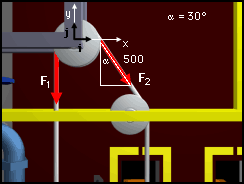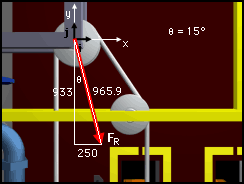Ch 2. Vectors Multimedia Engineering Statics Scalars & Vectors 2-DVectors 3-DVectors DotProducts
 Chapter 1. Basics 2. Vectors 3. Forces 4. Moments 5. Rigid Bodies 6. Structures 7. Centroids/Inertia 8. Internal Loads 9. Friction 10. Work & Energy Appendix Basic Math Units Sections Search eBooks Dynamics Fluids Math Mechanics Statics Thermodynamics Author(s): Kurt Gramoll ©Kurt GramollSTATICS - CASE STUDY SOLUTION Cartesian NotationCartesian Notation Diagram Choose an axis system as shown and write the two vectors in Cartesian notation. It is important that the correct sign is used.      F1 = 0i - 500j  lb      F2 = 500 sin30i - 500 cos30j  lb          = 250i - 433j  lb Addition of ForcesAddition of Forces Diagram Now that the two vectors are described using the unit vectors, i and j, they can been easily added. Adding the two forces will give the total force in Cartesian notation,      FR = F1 + F2 = 250i - (500 + 433)j          = 250i - 933j  lb The magnitude of the total force can be determined by squaring the i and j magnitudes, and then taking the square root,      FR = (2502 + 9332)0.5 = 965.9 lb The angle at which the total force acts is,      θ = tan-1 (250/933) = 15o

Practice Homework and Test problems now available in the 'Eng Statics' mobile app
Includes over 500 problems with complete detailed solutions.
Available now at the Google Play Store and Apple App Store.